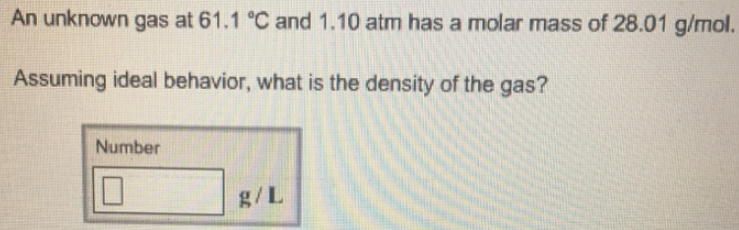# Problem: An unknown gas at 61.1 °C and 1.10 atm has a molar mass of 28.01 g/mol. Assuming ideal behavior, what is the density of the gas?

###### FREE Expert Solution
98% (372 ratings)###### Problem Details

An unknown gas at 61.1 °C and 1.10 atm has a molar mass of 28.01 g/mol. Assuming ideal behavior, what is the density of the gas?